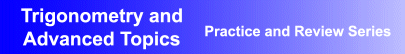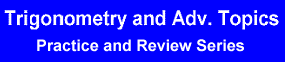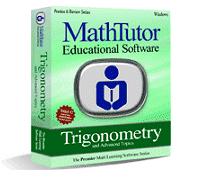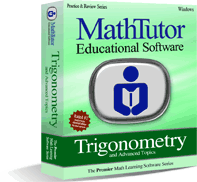Award-Winning

# Trigonometry Academic Instructional Review and Practice SoftwareDESCRIPTION
TRIGONOMETRY AND ADVANCED TOPICS is a highly regarded software package focused on drill, practice and review.
Designed by math educators, TRIGONOMETRY AND ADVANCED TOPICS provides a vast array of drill and practice exercises suitable for students at all levels of ability.
The program uses practice and drill problems to help solidify students' understanding of the concepts of trigonometry. Problems are based on students' skill levels and are generated using randomization algorithms.
TRIGONOMETRY AND ADVANCED TOPICS provides step by step explanations that help students build mastery in problem solving. Recordkeeping reports allow students to see the progress they have made.
Examples and problem solving exercises are based on student skill levels, allowing students to develop problem solving skills at a comfortable pace.
• Award-winning, curriculum-based trigonometry drill and practice software developed by math educators
• Interactive trigonometry problem solving exercises and explanations that boost student understanding of concepts
• Proven success helping students build proficiency in problem solvingTOPICS COVERED
Elementary Trigonometry
• Concepts and Definitions
• Right Triangle Trigonometry
• Elementary Identities I
• Basic Trigonometric Functions
• Quadrants of Angles
• Radians and Degrees
• Elementary Identities II
• Trigonometric Graphs I
• Angular Motion
• Elementary Identities III
• Trigonometric Graphs II
• Law of Sines and Cosines
• Word Probs: Law of Sines and Cosines
• Word Probs: Right Triangles
• Identities: Angle Addition and Subtr.
• Identities: Trigonometric
• Comprehensive Identities I
• Comprehensive Identities II
Vectors, Complex Nos., Exponents, Logs
• Vector Definitions
• Vector Applications
• Vector Components I
• Vector Components II
• Complex Numbers
• Exponents and Logs: Properties I
• Exponents and Logs: Graphs